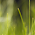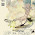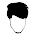# How to find GCD of two numbers in Java - Euclid's algorithm

How to find Greatest Common Divisor of two numbers in Java
Simple Java program to find GCD (Greatest common Divisor) or GCF  (Greatest Common Factor) or HCF (Highest common factor). The GCD of two numbers is the largest positive integer that divides both the numbers fully i.e. without any remainder. There are multiple methods to find GCD , GDF or HCF of two numbers but  Euclid's algorithm is very popular and easy to understand, of course, only if you understand how recursion works. Euclid's algorithm is an efficient way to find GCD of two numbers and it's pretty easy to implement using recursion in Java program. According to Euclid's method GCD of two numbers a, b is equal to GCD(b, a mod b) and GCD(a, 0) = a. The later case is the base case of our Java program to find GCD of two numbers using recursion. You can also calculate greatest common divisor in Java without using recursion but that would not be as easy as the recursive version, but still a good exercise from coding interviews point of view.

It's very easy to understand this algorithm once you look at the flow chart, which explains how Euclid's GCD algorithm works. You can also read Introduction to Algorithm by Thomas Cormen to learn more about similar computer algorithms.  This is one of the most popular books to learn Data structure and algorithms and widely used as textbooks for algorithms in many school, colleges, and universities. It is also popularly known as CLRS (Cormen, Leiserson, Rivest, Stein).

## GCD of two numbers in Java Code Example:

In Euclid's algorithm, we start with two numbers X and Y. If Y is zero then greatest common divisor of both will be X, but if Y is not zero then we assign the Y to X and Y becomes X%Y. Once again we check if Y is zero, if yes then we have our greatest common divisor or GCD otherwise we keep continue like this until Y becomes zero. Since we are using modulo operator, the number is getting smaller and smaller at each iteration, so the X%Y will eventually become zero.

Let' take an example of calculating GCD of 54 and 24 using Euclid's algorithm. Here X = 54 and Y = 24, since Y is not zero we move to the logical part and assign X = Y, which means X becomes 24 and Y becomes 54%24 i.e 6. Since Y is still not zero, we again apply the logic. This times X will become 6 and Y will become 24%6 i.e. Y=0. Bingo, Y is now zero which means we have our answer and it's nothing but the value of X which is 6 (six).

The algorithm will become clearer when you see the flow chart of calculating GCD of two numbers using recursion as shown below. You can see we are starting with two numbers X and Y and if Y=0 then we got our answer, otherwise, we apply logic and check again.  Now let's learn how to convert Euclid's algorithm to find GCD into Java code.

Here is my complete code example of how to find GCD of two numbers in Java. This Java program uses Euclid's method to find GCD of two numbers. Thy must be an integer, so make sure you check the numbers entered by user e.g. floating point numbers are not allowed.

Similarly, any alphabets and other characters are not allowed except '+' and '-' sign and all these rules are ensured by Scanner.nextInt() call. This method will throw an error if the user will enter an invalid value instead of an integer.

Java Program to calculate GCD of two numbers
```/**
* Java program to demonstrate How to find Greatest Common Divisor or GCD of
* two numbers using Euclid’s method. There are other methods as well to
* find GCD of two number in Java but this example of finding GCD of two number
* is most simple.
*
* @author Javin Paul
*/
public class GCDExample {

public static void main(String args[]){

//Enter two number whose GCD needs to be calculated.
Scanner scanner = new Scanner(System.in);
System.out.println("Please enter first number to find GCD");
int number1 = scanner.nextInt();
System.out.println("Please enter second number to find GCD");
int number2 = scanner.nextInt();

System.out.println("GCD of two numbers " + number1 +" and "
+ number2 +" is :" + findGCD(number1,number2));

}

/*
* Java method to find GCD of two number using Euclid's method
* @return GDC of two numbers in Java
*/
private static int findGCD(int number1, int number2) {
//base case
if(number2 == 0){
return number1;
}
return findGCD(number2, number1%number2);
}

}

Output:
Please enter first number to find GCD
54
Please enter second number to find GCD
24
GCD of two numbers 54 and 24 is :6```

That’s all on how to find GCD of two numbers in Java. You can use this Java program to prepare for viva or other computer homework and assignment test or for your self-practice to improve programming in Java. BTW, there are a couple of other technique to find Greatest common divisor in Java, as an exercise you can explore those methods and write code for that. The key point is you need to learn how to convert an algorithm into code to become a programmer.

If you like this little programming exercise and hungry for more to improve your coding skill, check out these exercises, they will help to build your programming logic :
• How to reverse String in Java without using API methods? (Solution)
• Write a function to find middle element of linked list in one pass? (solution)
• How to check if a number is binary in Java? (answer)
• Write a Program to Check if a number is Power of Two or not? (program)
• Write a method to check if two String are Anagram of each other? (method)
• Write a program to check if a number is Prime or not? (solution)
• Write a Program remove duplicates from an array without using Collection API? (program)
• Write a method to count occurrences of  a character in String? (Solution)
• How to find Fibonacci sequence up to a given Number? (solution)
• How to check if LinkedList contains any cycle in Java? (solution)
• How to check if a number is Armstrong number or not? (solution)
• How to find largest and smallest number in an array? (solution)
• Write a method to remove duplicates from ArrayList in Java? (Solution)
• How to solve Producer Consumer Problem in Java. (solution)
• How to find prime factors of an integer in Java? (solution)
• Write a program to find first non-repeated characters from String in Java? (program)

#### 13 comments:

1.Can you also share program to find LCD for numbers in Java? I understand calculating GCD using Euclid's method, but don't find any short trick for calculating LCD similarly. Please help

1.LCD=(n1*n2)/GCD

2.What is the other way of calculating GCD in Java program, apart from Euclid's method, a comparison would be nice.

3.private static int findlcm(int number1, int number2) {
int lcm=0;
for(int i=1;i<=number2;i++){
lcm=number1*i;
if(lcm%number2==0){
break;
}
}
return lcm;
}

1.i built a program w/c is suppose to calculate GCD and LCD but i dont know where to insert its formula??
here is my code..

import java.awt.*;
import java.awt.event.*;
import javax.swing.*;

public class Distruc extends JFrame implements ActionListener{

Container con;
JLabel lbl1,lbl2,lbl3;
JTextField txt1,txt2,txt3;
JButton btn1,btn2,btn3;

public Distruc(){
con = getContentPane();
con.setLayout(new FlowLayout());

txt1 = new JTextField(10);
txt2 = new JTextField(10);
txt3 = new JTextField(10);

lbl1 = new JLabel("INPUT 1: ");
lbl2 = new JLabel("INPUT 2: ");
lbl3 = new JLabel("RESULT: ");

btn1 = new JButton("GCD");
btn1.addActionListener(this);
btn2 = new JButton("LCD");
btn2.addActionListener(this);
btn3 = new JButton("DONE");
btn3.addActionListener(this);

txt1.setText("");
txt2.setText("");
txt3.setText("");

con.add(lbl1);
con.add(txt1);
con.add(lbl2);
con.add(txt2);
con.add(btn1);
con.add(btn2);
con.add(lbl3);
con.add(txt3);
con.add(btn3);

}

public void actionPerformed(ActionEvent e){
try{
Object sc = e.getSource();

String a = txt1.getText();
String b = txt2.getText();

if(sc==btn1){

}
else if(sc==btn2){

}
else if(sc==btn3){
System.exit(0);
}
}
catch(NumberFormatException error){
JOptionPane.showMessageDialog(null, "Error");

}
}
public static void main(String[]args){

Distruc dt = new Distruc();

dt.setSize(170,240);
dt.setVisible(true);
dt.setResizable(false);
dt.setTitle("Distruc");
dt.setLocationRelativeTo(null);
dt.setDefaultCloseOperation(JFrame.EXIT_ON_CLOSE);
}
}

pls help...

4.plz tell me gcd program via factorial

5.plz help me with this....Wap in Java to input two numbers in LCM and GCM make use of the function
1.void take(int a,int b)-to take the value of a and b.
2.void calculate-calculate GCD and LCM.
3.void show-display output.

6.Please help with recursive method for lcm

7.nice...thnx...i think it gave me only one error where i had to import scanner separately...

8.Can you show the code without a recursion method

9.The flowchart is wrong. After x=y then x%y = 0 always. Your program is simultaneously resetting x and y with the same original x and y for each reset.

10.logic above takes n1 > n2, so n1 & n2 should be swapped if n1 < n2

11.This code is super cool!!

Can anyone do this with array of integers that the user can give inputs at the console?